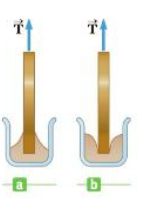Chapter 9, Problem 49P

Chapter
Section
Textbook Problem

Surface Tension, Capillary Action, and Viscous Fluid Flow49. A square metal sheet 3.0 cm on a side and of negligible thickness is attached to a balance and inserted into a container of fluid. The contact angle is found to be zero, as shown in Figure P9.49a, and the balance to which the metal sheet is attached reads 0.40 N. A thin veneer of oil is then spread over the sheet, and the contact angle becomes 180°, as shown in Figure P9.49b. The balance now reads 0.39 N. What is the surface tension of the fluid?Figure P9.49

To determine
The surface tension of the fluid.

Explanation
The sum of vertical forces on the square metal sheet is Fy=TmgFy=0T=mg+Fy . The vertical component of the surface tension force Fy=γ(2L)cosϕ since the force is two sided. Now the force of tension is modified as T=mg+γ(2L)cosϕ . Use the conditions when ϕ=0° the force of tension would be T1=mg+γ2L and T2=mg2γL for the angle contact ϕ=180° . These two equations of tensions, the surface tension of the fluid is determined.

Given info: The side of the square metal sheet is 3.0cm The tensions on the square metal are 0.40N and 0.39N .

The formula for the surface tension of the fluid is,

γ=T1T24L

• T1 and T2 are tensions

Still sussing out bartleby?

Check out a sample textbook solution.

See a sample solution

The Solution to Your Study Problems

Bartleby provides explanations to thousands of textbook problems written by our experts, many with advanced degrees!

Get Started